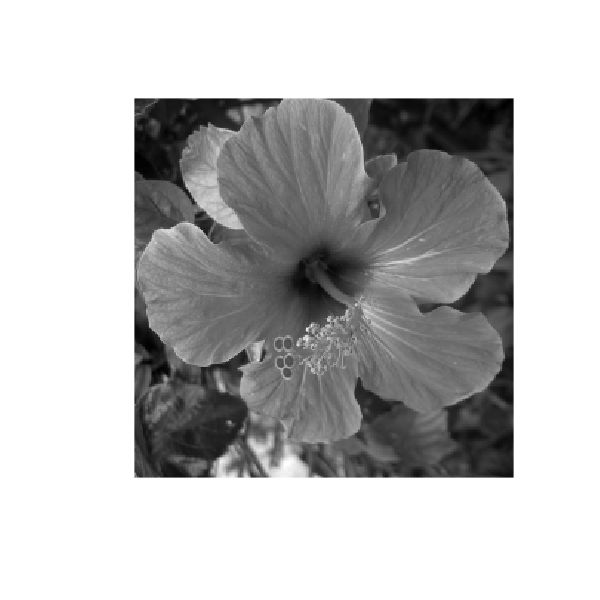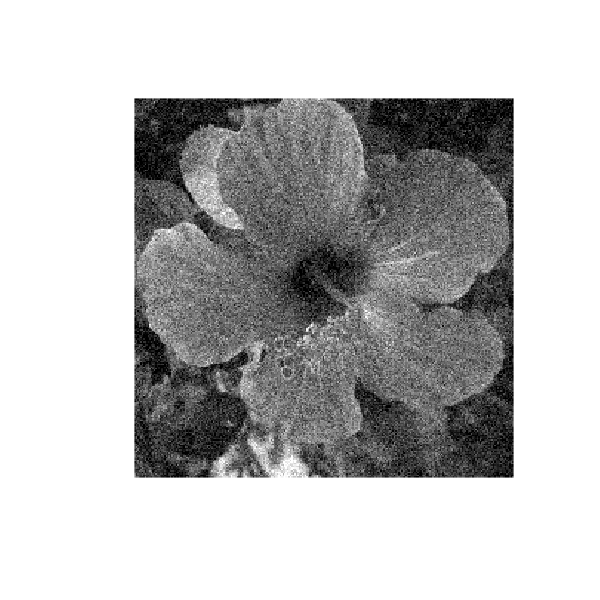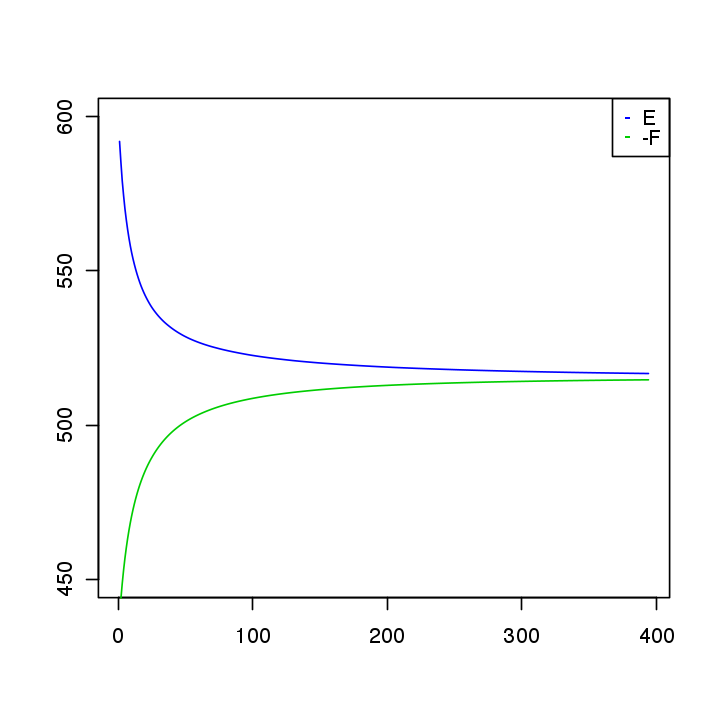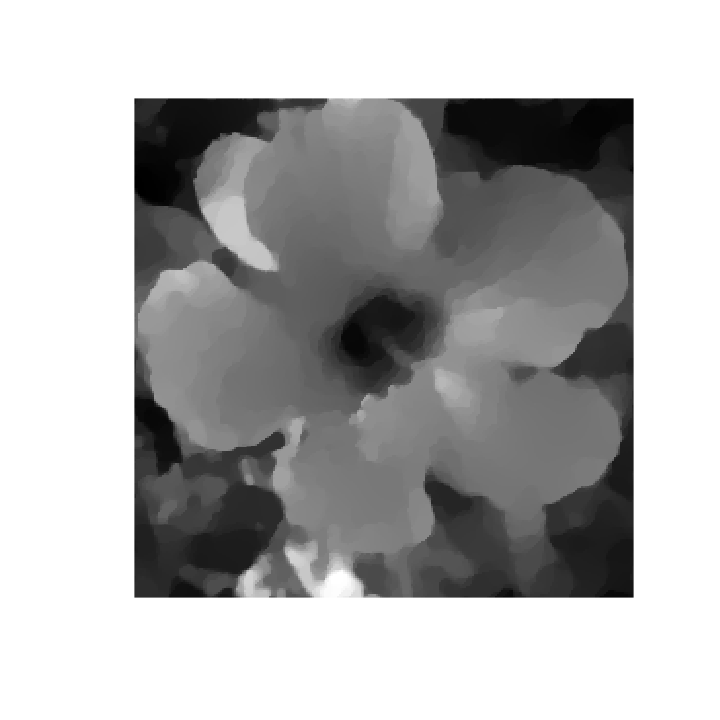# Fenchel-Rockafellar Duality¶

Important: Please read the installation page for details about how to install the toolboxes. $\newcommand{\dotp}{\langle #1, #2 \rangle}$ $\newcommand{\enscond}{\lbrace #1, #2 \rbrace}$ $\newcommand{\pd}{ \frac{ \partial #1}{\partial #2} }$ $\newcommand{\umin}{\underset{#1}{\min}\;}$ $\newcommand{\umax}{\underset{#1}{\max}\;}$ $\newcommand{\umin}{\underset{#1}{\min}\;}$ $\newcommand{\uargmin}{\underset{#1}{argmin}\;}$ $\newcommand{\norm}{\|#1\|}$ $\newcommand{\abs}{\left|#1\right|}$ $\newcommand{\choice}{ \left\{ \begin{array}{l} #1 \end{array} \right. }$ $\newcommand{\pa}{\left(#1\right)}$ $\newcommand{\diag}{{diag}\left( #1 \right)}$ $\newcommand{\qandq}{\quad\text{and}\quad}$ $\newcommand{\qwhereq}{\quad\text{where}\quad}$ $\newcommand{\qifq}{ \quad \text{if} \quad }$ $\newcommand{\qarrq}{ \quad \Longrightarrow \quad }$ $\newcommand{\ZZ}{\mathbb{Z}}$ $\newcommand{\CC}{\mathbb{C}}$ $\newcommand{\RR}{\mathbb{R}}$ $\newcommand{\EE}{\mathbb{E}}$ $\newcommand{\Zz}{\mathcal{Z}}$ $\newcommand{\Ww}{\mathcal{W}}$ $\newcommand{\Vv}{\mathcal{V}}$ $\newcommand{\Nn}{\mathcal{N}}$ $\newcommand{\NN}{\mathcal{N}}$ $\newcommand{\Hh}{\mathcal{H}}$ $\newcommand{\Bb}{\mathcal{B}}$ $\newcommand{\Ee}{\mathcal{E}}$ $\newcommand{\Cc}{\mathcal{C}}$ $\newcommand{\Gg}{\mathcal{G}}$ $\newcommand{\Ss}{\mathcal{S}}$ $\newcommand{\Pp}{\mathcal{P}}$ $\newcommand{\Ff}{\mathcal{F}}$ $\newcommand{\Xx}{\mathcal{X}}$ $\newcommand{\Mm}{\mathcal{M}}$ $\newcommand{\Ii}{\mathcal{I}}$ $\newcommand{\Dd}{\mathcal{D}}$ $\newcommand{\Ll}{\mathcal{L}}$ $\newcommand{\Tt}{\mathcal{T}}$ $\newcommand{\si}{\sigma}$ $\newcommand{\al}{\alpha}$ $\newcommand{\la}{\lambda}$ $\newcommand{\ga}{\gamma}$ $\newcommand{\Ga}{\Gamma}$ $\newcommand{\La}{\Lambda}$ $\newcommand{\si}{\sigma}$ $\newcommand{\Si}{\Sigma}$ $\newcommand{\be}{\beta}$ $\newcommand{\de}{\delta}$ $\newcommand{\De}{\Delta}$ $\newcommand{\phi}{\varphi}$ $\newcommand{\th}{\theta}$ $\newcommand{\om}{\omega}$ $\newcommand{\Om}{\Omega}$

In :
library(pracma)
library(imager)

options(warn=-1) # turns off warnings, to turn on: "options(warn=0)"

# Importing the libraries
for (f in list.files(path="nt_toolbox/toolbox_general/", pattern="*.R")) {
source(paste("nt_toolbox/toolbox_general/", f, sep=""))
}
for (f in list.files(path="nt_toolbox/toolbox_signal/", pattern="*.R")) {
source(paste("nt_toolbox/toolbox_signal/", f, sep=""))
}


This numerical tour is an introduction to convex duality with an application to total variation denoising.

## Convex Duality¶

Given some convex, proper, and lower semi-continuous function $f(x)$ defined for $x \in \RR^N$, its Legendre-Fenchel dual function is defined as $$\forall u \in \RR^N, \quad f^*(u) = \umax{x \in \RR^N} \dotp{x}{u} - f(x).$$

One can show that $f^*$ is a convex function, and that it satisfies $(f^*)^* = f$.

One can show if $f(x) = \frac{1}{2} \norm{A x - b}^2$ where $A \in \RR^{N \times N}$ is an invertible matrix, then $$f^*(u) = \frac{1}{2} \norm{\tilde A u + b}^2 \qwhereq \tilde A = (A^*)^{-1}.$$

One can show that in the case of $\ell^p$ norms $$f(x) = \norm{x}_p = \pa{ \sum_{i=1}^N \abs{x_i}^p }^{1/p}$$ with the usual extension to $p=+\infty$ $$\norm{x}_\infty = \umax{1 \leq i \leq N} \abs{x_i}$$ then one has $$f^*(u) = \iota_{\norm{\cdot}_q \leq 1} \qwhereq \frac{1}{p}+\frac{1}{q}=1,$$ where $\iota_{\Cc}$ is the indicator function of the convex set $\Cc$.

## FB on the Fenchel-Rockafellar Dual Problem¶

We are concerned with the minimization of composite problems of the form $$\umin{x \in \RR^N} f(x) + g(A(x))$$ where $A \in \RR^{P \times N}$ is a linear map (a matrix), $f : \RR^N \rightarrow \RR$ and $g : \RR^P \rightarrow \RR$ are convex functional.

We now assume that $f$ is a $L$-strongly convex function. In this case, one can show that $f^*$ is a $C^1$ smooth function, and that its gradient is $L$-Lipschitz.

In this case, the Fenchel-Rockafellar theorem shows that one can solve the following dual problem $$\umin{x \in \RR^N} f(x) + g(A(x)) = • \umin{u \in \RR^P} f^( -A^ u ) + g^(u)$$ and recover the unique solution $x^\star$ of the primal problem from a (non-necessarily unique) solution $u^\star$ to the dual problem as $$x^\star = \nabla f^( -A^* u^\star ).$$

Denoting $F(u) = f^*( -A^* u )$ and $G(u) = g^*(u)$, one thus needs to solve the problem $$\umin{u \in \RR^P} F(u) + G(u).$$

We assume that the function $g$ is simple, in the sense that one can compute in closed form the so-called proximal mapping, which is defined as $$\text{prox}_{\ga g}(x) = \uargmin{z \in \RR^N} \frac{1}{2}\norm{x-z}^2 + \ga g(z).$$ for any $\ga > 0$.

Note that $g$ being simple is equivalent to $g^*$ also being simple because of Moreau's identity: $$x = \text{prox}_{\tau g^*}(x) + \tau \text{prox}_{g/\tau}(x/\tau).$$

Since $F$ is smooth and $G$ is simple, one can apply the Foward-Backward algorithm, which reads, after initializing $u^{(0)} \in \RR^P$, $$u^{(\ell+1)} = \text{prox}_{\ga G}\pa{ u^{(\ell)} - \ga \nabla F( u^{(\ell)} ) }.$$ with $\ga < 2/L$.

The primal iterates are defined as $$x^{(\ell)} = \nabla F( -A^* u^{(\ell)} ).$$

## Total Variation¶

The total variation of a smooth function $\phi : \RR^2 \rightarrow \RR$ is defined as $$J(\phi) = \int \norm{\nabla \phi(s)} d s$$ The total variation of an image is also equal to the total length of its level sets. $$J(\phi) = \int_{-\infty}^{+\infty} L( S_t(\phi) ) dt.$$ Where $S_t(\phi)$ is the level set at $t$ of the function $\phi$ $$S_t(\phi)= \enscond{ s }{ \phi(s)=t } .$$ This shows that the total variation can be extended to functions having step discontinuities.

We consider images $x = (x_{i,j})_{i,j} \in \RR^N$ of $N=n\times n$ pixels.

We consider here a discretized gradient operator $A : \RR^N \rightarrow \RR^P$ where $P=2N$ defined as $$A x = u = (u^1,u^2) \qwhereq u^1 = ( x_{i+1,j}-x_{i,j} )_{ i,j } \in \RR^N \qandq u^2 = ( x_{i,j+1}-x_{i,j} )_{ i,j } \in \RR^N.$$ where we assume periodic boundary conditions for simplicity.

The adjoint $A^*$ of the discrete gradient is minus the discrete divergence.

In :
# Renaming the imported div_2 and grad_2 function
div = div_2

As = function(u){-div(u)}


In the following, while images $x \in \RR^{N}$ are stored as arrays of size |(n,n)|, gradient vector fields $u \in \RR^P$ are stored as arrays size |(n,n,2)|.

The discrete total variation is defined as the $\ell^1-\ell^2$ norm of the discretized gradient $$J(x) = \norm{A x}_{1,2} \qwhereq \norm{u}_{1,2} = \sum_{i,j} \norm{u_{i,j}}$$ where $u = (u_{i,j} \in \RR^2)_{i,j}$ is a vector field.

In :
norm12 = function(u){sum(sum(sqrt((u^2)[,,1] + (u^2)[,,2])))}
J = function(x){norm12(A(x))}


## Total Variation Regularization¶

We consider here denoising using total variation regularization. This was first introduced in:

L.I. Rudin, S. Osher, E. Fatemi, Nonlinear total variation based noise removal algorithms, Physica D, vol. 60, pp. 259-268, 1992.

Given a noisy image $y \in \RR^N$, it computes $$x^\star = \uargmin{x \in \RR^N} \frac{1}{2}\norm{x-y}^2 + \la J(x),$$ where the regularization parameter $\la \geq 0$ should be adapted to the noise level.

Number of pixels.

In :
n = 256


First we load an image $x_0 \in \RR^N$ of $N=n \times n$ pixels.

In :
name = "nt_toolbox/data/hibiscus.png"
x0 = load_image(name, n)
x0 = resize(x0, size_x=n, size_y=n)
# Two dimensional
x0 = x0[,,1,1]


Display the original image $x_0$.

In :
options(repr.plot.width=5, repr.plot.height=5)
imageplot(clamp(x0))Add some noise to the original image, to obtain $y=x_{0}+w$.

In :
sigma = 0.1
set.seed(1)
y = x0 + randn(n,n) * sigma


Display the noisy image $y$.

In :
imageplot(clamp(y))Set the regularization parameter $\la$.

In :
lambda = 0.2


## Chambolle Dual Algorithm ¶

We consider here the application of FB on the dual of the ROF problem, as initially proposed in:

Antonin Chambolle, An Algorithm for Total Variation Minimization and Applications, Journal of Mathematical Imaging and Vision, 20(1-2), 2004.

An earlier version of this algorithm was proposed in:

B. Mercier, Inequations Variationnelles de la Mecanique Publications Mathematiques d'Orsay, no. 80.01. Orsay, France, Universite de Paris-XI, 1980.

For a description of a more general framework, see:

P. L. Combettes, Dinh Dung, and B. C. Vu, Dualization of signal recovery problems, Set-Valued and Variational Analysis, vol. 18, pp. 373-404, December 2010

The primal problem corresponds to minimizing $E(x) = f(x)+g(A(x))$ where $$f(x) = \frac{1}{2}\norm{x-y}^2 \qandq g(u) = \la \norm{u}_{1,2}.$$

In :
f = function(x){0.5 * norm(x-y)^2}
g = function(x){lambda * J(x)}
E = function(x){f(x) + g(x)}


The dual problem corresponds to minimzing $F(u)+ G(u)$ where $$F(u) = \frac{1}{2} \norm{y - A^* u}^2 - \frac{1}{2}\norm{y}^2 \qandq G(u) = \iota_{\Cc}(u) \qwhereq \Cc = \enscond{u}{\norm{u}_{\infty,2} \leq \la}.$$ where $$\norm{u}_{\infty,2} = \umax{i,j} \norm{u_{i,j}}$$

In :
F = function(u){0.5 * norm(y - As(u))^2 - 0.5 * norm(y)^2}


One can thus solves the ROF problem by computing $$x^\star = y - A^* u^\star$$ where $$u^\star \in \uargmin{ \norm{u}_{1,2} \leq \la } \norm{y - A^* u}$$

One can compute explicitely the gradient of $F$: $$\nabla F(u) = A (A^* u - y).$$

In :
nablaF = function(u){A(As(u) - y)}


The proximal operator of $G$ is the orthogonal projection on $\Cc$, which is obtained as $$\text{prox}_{\ga G}(u)_{i,j} = \frac{u_{i,j}}{ \max(1,\norm{u_{i,j}}/\lambda) }.$$ Note that it does not depends on $\ga$.

In :
d = function(u)
{
n = dim(u)
p = dim(u)

out = array(0, c(n, p, 2))

for (i in 1:n)
{
for (j in 1:p)
{
out[i, j,] = norm(u[i, j,])
}
}
return (out)
}

proxG = function(u){u / pmax(d(u) / lambda, 1)}


The gradient step size of the FB should satisfy $$\ga < \frac{2}{\norm{A^* A}} = \frac{1}{4}.$$

In :
gamma = 1./5


Initialize the FB with $u=0 \in \RR^P$.

In :
u = array(0, c(n, n, 2))


One step of FB.

In :
u = proxG(u - gamma * nablaF(u))


Update the solution using $$x^{(\ell)} = y - A^* u.$$

In :
x = y - As(u)


Exercise 1

Perform Chambolle algorithm to solve the ROF problem. Monitor the primal $E$ and dual $-F$ energies.

In :
source("nt_solutions/optim_7_duality/exo1.R")In :
# Insert your code here.


Display the denoised image $x^\star$.

In :
imageplot(clamp(x))